Processing ......FreeComputerBooks.com Links to Free Computer, Mathematics, Technical Books all over the World

Computational Complexity
Related Book Categories:
•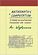Mathematics and Computation (Avi Wigderson)

This book provides a broad, conceptual overview of computational complexity theory - the mathematical study of efficient computation. With important practical applications to computer science and industry, computational complexity theory, etc.

•Think Complexity: Science and Modeling, 2nd Ed. (Allen Downey)

This book is about complexity science, data structures and algorithms, intermediate programming in Python, and the philosophy of science. It presents features that make Python such a simple and powerful language. Code with a solution for each exercise.

•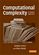Complexity Theory: A Modern Approach (Sanjeev Arora, et al)

This beginning graduate textbook describes both recent achievements and classical results of computational complexity theory, including interactive proofs, PCP, derandomization, and quantum computation.

•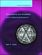Computability and Complexity: From a Programming Perspective

This book is an introduction to the basic concepts of computability, complex, and the theory of programming languages. Its goal is to build a bridge between computability and complexity theory and other areas of computer science, especially programming.

•Quantum Computing Since Democritus (Scott Aaronson)

This book takes readers on a tour through some of the deepest ideas of maths, computer science and physics. Full of insights, arguments and philosophical perspectives, the book covers an amazing array of topics.

•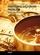Traveling Salesman Problem, Theory and Applications

This book is a collection of current research in the application of evolutionary algorithms and other optimal algorithms to solving the TSP problem, including Artificial Immune Systems, Genetic Algorithms, Neural Networks and Differential Evolution Algorithm.

•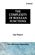The Complexity of Boolean Functions (Ingo Wegener)

Initially deals with the wee-known computation models, and goes on to special types of circuits, parallel computers, and branching programs. It presents a large number of recent research results f Boolean functions previously unavailable in book form.

•Computational Complexity (Wikibooks)

This book contains material that should be core knowledge in the theory of computation for all graduates in computer science. It starts with classical computability theory which forms the basis for complexity theory.

•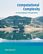Computational Complexity: A Conceptual Perspective (Goldreich)

This book offers a conceptual introduction to the study of the intrinsic complexity of computational tasks. It is intended to serve advanced undergraduate and graduate students, either as a textbook or for self-study.

•Algorithms and Complexity (Herbert S. Wilf)

This is an introductory book on the design and analysis of algorithms. The author uses a careful selection of a few topics to illustrate the tools for algorithm analysis.

•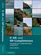P, NP, and NP-Completeness: The Basics of Complexity

The book starts with the relevant background in computability, including Turing machines, search and decision problems, algorithms, circuits, and complexity classes, and then focuses on the P-versus-NP Question and the theory of NP-completeness.

•Cellular Automata And Complexity (Stephen Wolfram)

This volume seems to be interesting for computer scientists, especially for those engaged in parallelization, and for physicists and chemists having to discover the basic rules of complex systems (by cellular computer simulation)

•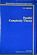Parallel Complexity Theory (Ian Parberry)

This book's aim is to show how the theoretical study of parallel computing complexity can be useful in the process of designing effective parallel algorithms.

•Computational Modeling and Complexity Science: Python Edition

This book is about complexity science, data structures and algorithms, intermediate programming in Python, and the philosophy of science.

•Models of Computation: Exploring the Power of Computing

It covers the traditional topics of formal languages, automata and complexity classes, as well as an introduction to the more modern topics of space-time tradeoffs, memory hierarchies, parallel computation, the VLSI model, and circuit complexity.

•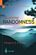Exploring Randomness (Gregory J. Chaitin)

This book presents the technical core of the theory of program-size complexity. LISP is used to present the key algorithms and to enable computer users to interact with the authors proofs and discover for themselves how they work.

•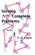Solving NP-Complete Problems (Forbes D. Lewis)

This is a textbook on heuristic algorithms. From the table of contents: Classes of Problems; Integer Programming; Enumeration Techniques; Dynamic Programming; Approximate Solutions; Local Optimization; Natural Models.

Book Categories
 :All CategoriesRecent BooksMiscellaneous BooksComputer LanguagesComputer ScienceData Science/DatabasesElectrical EngineeringJava and Java EE (J2EE)Linux and UnixMathematicsMicrosoft and .NETMobile ComputingNetworking and CommunicationsSoftware EngineeringSpecial TopicsWeb Programming
Other Categories